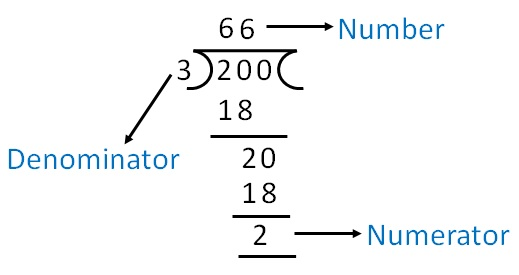Converting fraction to percent

Chapter 7 Class 7 Comparing Quantities
Concept wise

Suppose we have fraction

1/2

We want to convert it into percentage

How do we do it?

We know that 1/100 = 1%

So, we multiply & divide the fraction by 100

Thus,

1/2  = 1/2 × 100/100

= 100/2 × 1/100

= 100/2 × %

= 50%

We can also write directly

1/2  = 1/2 × 100 %

= 100/2 %

= 50%

#### Convert 9/8 into percent.

9/8 = 9/8 × 100%

= 9 × 100/8 %

= 9 × 50/4 %

= 9 × 25/2 %

= 225/2 %

= 112.5%

#### Convert 2/3 into percent.

2/3 = 2/3 × 100%

= 200/3 %

= 66 2/3 %Learn in your speed, with individual attention - Teachoo Maths 1-on-1 Class